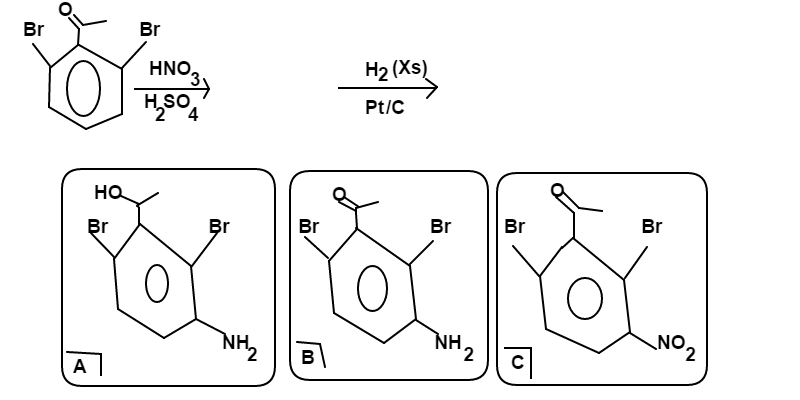# 2,6-dibromo Acetophenone Nitrogenation

Chemistry Level 3$\ce{2,6-dibromo~acetophenone}$ is reacted in the following order:

• $\ce{HNO3}$ in $\ce{H_2SO_4}$.

• $\ce{H_2}$ in excess ($Xs$) with $Pt/C$.

From among choices $A$, $B$, and $C$; choose the major product of this reaction scheme. If the major product is not among the choices, choose $\ce{No~Reaction}$ as your answer.

###### David's Physical Chemistry Set
×

Problem Loading...

Note Loading...

Set Loading...